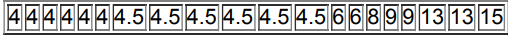×
Get Full Access to Elementary Statistics - 12 Edition - Chapter 3.3 - Problem 15bsc
Get Full Access to Elementary Statistics - 12 Edition - Chapter 3.3 - Problem 15bsc

×

# In Exercises, find the range, variance, and | Ch 3.3 - 15BSCISBN: 9780321836960 18

## Solution for problem 15BSC Chapter 3.3

Elementary Statistics | 12th Edition

• Textbook Solutions
• 2901 Step-by-step solutions solved by professors and subject experts
• Get 24/7 help from StudySoup virtual teaching assistantsElementary Statistics | 12th Edition

4 5 1 260 Reviews
19
0
Problem 15BSC

Years to Earn Bachelor's Degree Listed below are the lengths of time (in years) it took for a random sample of college students to earn bachelor's degrees (based on data from the National Center for Education Statistics). Based on these results, is it unusual for someone to earn a bachelor's degree in 12 years?Step-by-Step Solution:

Step 1 of 1 :

Mercury in Sushi Listed below are the amounts of mercury (in parts per million, or ppm) found in tuna sushi sampled at different stores in New York City. The study was sponsored by the New York Times, and the stores (in order) are D’Agostino, Eli’s Manhattan, Fairway, Food Emporium, Gourmet Garage, Grace’s Marketplace, and Whole Foods.

Given data

0.56 , 0.75 , 0.10 , 0.95 , 1.25 , 0.54 , 0.88

Now we have to find the range, variance, and standard deviation for the given sample data.

Range :

The range is a simple measure of variation in a set of random variables. It is difference between the biggest and smallest random variable.

Range = Maximum value - Minimum value

Given data

0.56 , 0.75 , 0.10 , 0.95 , 1.25 , 0.54 , 0.88

Therefore, the range of the seven random variables 0.56 , 0.75 , 0.10 , 0.95 , 1.25 , 0.54 , 0.88

Range = Maximum value - Minimum value

Range = 1.25 - 0.1

Range = 1.15 mm

Sample variance :

The variance of a sample is defined by slightly different formula:

The sample variance is the square difference of the data value to the mean divided by the number of values.

s2 =where s2 is the sample variance.

x is the sample mean.

xi is the ith element from the sample and

n is the number of elements in the sample.= 0.56 + 0.75 + 0.10 + 0.95 + 1.25 + 0.54 + 0.88 = 0.7186= 0.7186

s2 =Therefore variance s2 = 0.133 .

The standard deviation is the square root of the sample variance.

s =s =s0.366

If the tuna sushi contained no mercury, all of the measures would be 0 ppm, and the measures of variation would all be 0 as well.

Step 2 of 1

##### ISBN: 9780321836960

Since the solution to 15BSC from 3.3 chapter was answered, more than 573 students have viewed the full step-by-step answer. This textbook survival guide was created for the textbook: Elementary Statistics, edition: 12. Elementary Statistics was written by and is associated to the ISBN: 9780321836960. This full solution covers the following key subjects: mercury, measures, sushi, new, stores. This expansive textbook survival guide covers 121 chapters, and 3629 solutions. The answer to “?Years to Earn Bachelor's Degree Listed below are the lengths of time (in years) it took for a random sample of college students to earn bachelor's degrees (based on data from the National Center for Education Statistics). Based on these results, is it unusual for someone to earn a bachelor's degree in 12 years?” is broken down into a number of easy to follow steps, and 54 words. The full step-by-step solution to problem: 15BSC from chapter: 3.3 was answered by , our top Statistics solution expert on 03/15/17, 10:30PM.

## Discover and learn what students are asking

Calculus: Early Transcendental Functions : Space Coordinates and Vectors in Space
?In Exercises 1-4, plot the points in the same three-dimensional coordinate system. (a) (3, -2, 5)

Unlock Textbook Solution